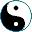<<
>>
A monad in category C is an endofunctor F: CC with two natural transformations:
u: 1 → F and m: F ∘ F → F.
Let's denote F(u): F -> F ∘ F the transformation that results in applying F to u, and F(m) : (F ∘ F) ∘ F → F ∘ F.
Having these, now we can write down the following two monad axioms:

1. F(u) ∘ m is identity F → F
2. :
 F(X) F(uX) F(F(X)) mF(X) F(X) → →

3. F(m) ∘ m is the same as m ∘ m:
 F(F(F(X))) F(mX) F(F(X)) —→ mF(X)↓ ↓mX F(F(X)) —→ F(X) mX